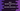# 3 ways to convert System.nanoTime to seconds in Java## How to convert System.nanoTime to seconds in Java:

In this post, we will learn how to convert System.nanoTime to seconds in Java in different ways. The System.nanoTime method is used to get the current value of the JVM’s time source in nanoseconds. The return value of System.nanoTime should only be used to find elapsed time. It is not related to system time.

### System.nanoTime:

The nanoTime is a static method defined in the System class:

``public static native long nanoTime();``

It returns the time in long format.

This method is used mostly to find out the execution time in code. We can calculate this value in two places and find the difference to get the execution time between the two points. Let’s learn how to convert the nanoseconds value to seconds in different ways in Java with examples.

### Method 1: By division:

We know that 1 second is equal to 1000000000 nanoseconds. So, if we divide the nanoseconds value by 1000000000, it will return the value in seconds.

``````public class Main {
public static void main(String[] args) {
long startTime = System.nanoTime();
try {
} catch (InterruptedException e) {
throw new RuntimeException(e);
}
long timeDiff = System.nanoTime() - startTime;
double timeDiffInSeconds = (double) timeDiff / 1_000_000_000;

System.out.println("Time difference in nanoseconds: " + timeDiff);
System.out.println("Time difference in seconds: " + timeDiffInSeconds);
}
}``````

In this example, we captured the time at the start of the program. The program is halted for 10 seconds by using Thread.sleep. It captured the time again and time difference is calculated.

The variable time is used to hold the time difference in nanoseconds. We divided this time by 1000000000 to find the difference in seconds and the value is assigned to timeDiffInSeconds variable.

If you run this program, it will print:

``````Time difference in nanoseconds: 10004324181
Time difference in seconds: 10.004324181``````

### Method 2: By using TimeUnit.toSeconds():

The TimeUnit class provides a method toSeconds to convert a value to seconds. We can use this method to convert a nanoseconds value to seconds.

This method returns the converted value in long format.

``public long toSeconds(long duration)``

Let me show you how it works with an example:

``````import java.util.concurrent.TimeUnit;

public class Main {
public static void main(String[] args) {
long startTime = System.nanoTime();
try {
} catch (InterruptedException e) {
throw new RuntimeException(e);
}
long timeDiff = System.nanoTime() - startTime;
long timeDiffInSeconds = TimeUnit.NANOSECONDS.toSeconds(timeDiff);

System.out.println("Time difference in nanoseconds: " + timeDiff);
System.out.println("Time difference in seconds: " + timeDiffInSeconds);
}
}``````

This is similar to the first example. I am using TimeUnit.NANOSECONDS.toSeconds method to convert the nanoseconds value to seconds.

### Method 3: By using TimeUnit.convert():

There is one more method in the TimeUnit class called convert() for time conversion. We can use it to convert the nanoseconds time to seconds. This method takes two parameters:

``public long convert(long sourceDuration, TimeUnit sourceUnit)``

The first parameter is the time in long that we wants to convert. The second parameter is the unit of the source time that we are converting. It returns the converted time in long format.

``````import java.util.concurrent.TimeUnit;

public class Main {
public static void main(String[] args) {
long startTime = System.nanoTime();
try {
} catch (InterruptedException e) {
throw new RuntimeException(e);
}
long timeDiff = System.nanoTime() - startTime;
long timeDiffInSeconds = TimeUnit.SECONDS.convert(timeDiff, TimeUnit.NANOSECONDS);

System.out.println("Time difference in nanoseconds: " + timeDiff);
System.out.println("Time difference in seconds: " + timeDiffInSeconds);
}
}``````

We are passing the time difference in nanoseconds as the first parameter and TimeUnit.NANOSECONDS as the second parameter. This method returns the time in seconds:

``````Time difference in nanoseconds: 10003272169
Time difference in seconds: 10``````